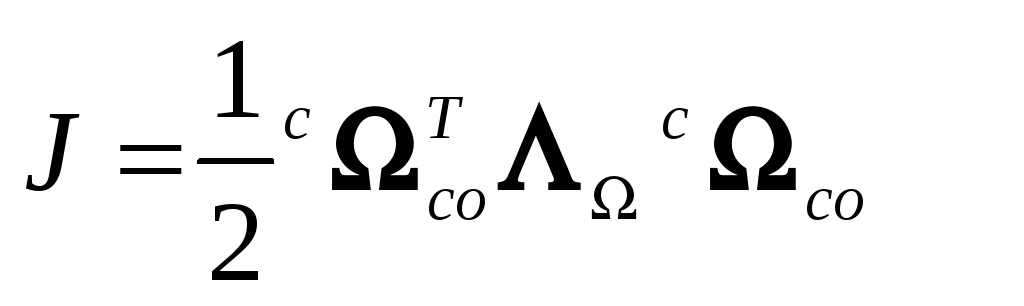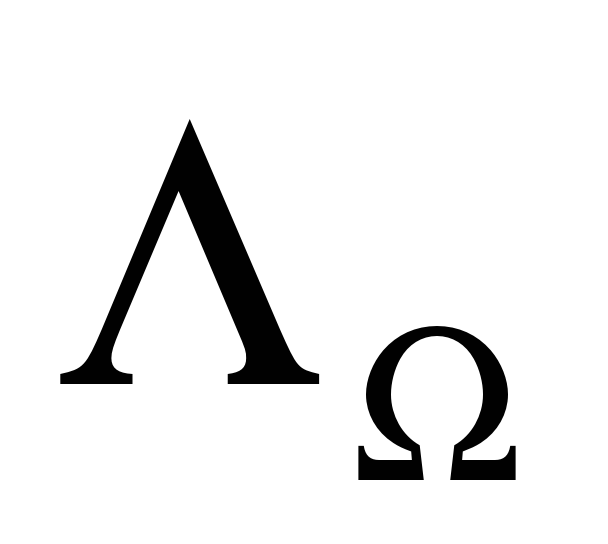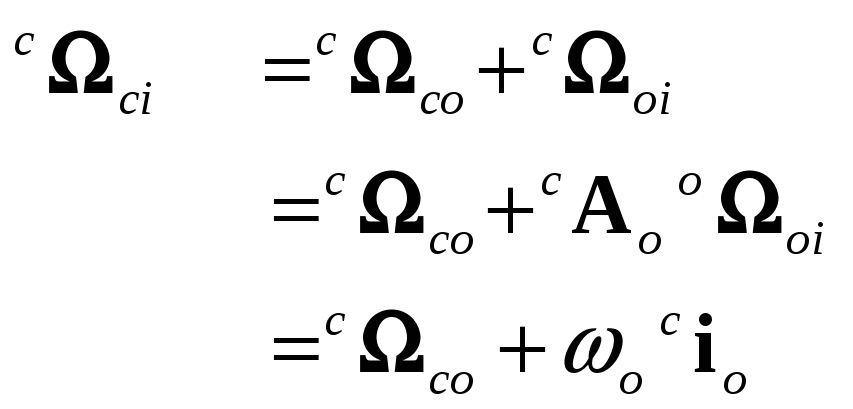# Pedro Tavares, Paulo Tabuada, ‡

 Formulation As in (Steyn, 1994), the measurements of the current geomagnetic field and satellite angular velocity are used to determine the control magnetic moment. We start by defining a cost function based on the kinetic energy1:(5) whereis a positive definite gain matrix. More insight will be given regarding the choice of the cost function, when studying the algorithm stability in Stability Study sub-section. The dynamic model of the satellite is well known and understood so it can be used to see the influence of the magnetic moment on the angular velocity. The angular velocity of the Control CS w.r.t. the Inertial CS can be written as:(6) Download 1.52 Mb.Share with your friends: